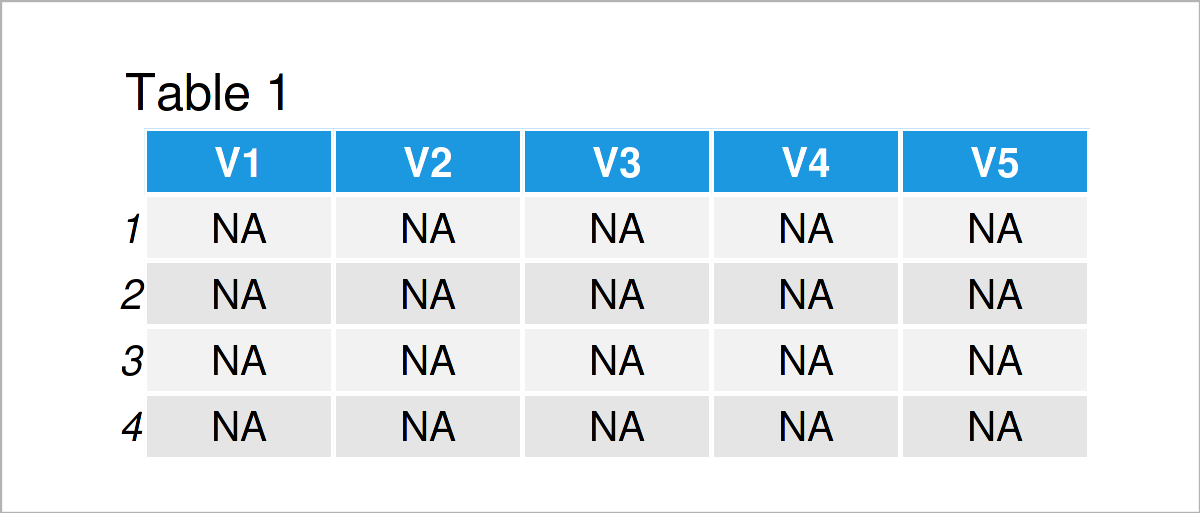# Create Empty data.table with Column Names in R (2 Examples)

This article shows how to build a blank data.table including column names in the R programming language. For general information regarding data.tables in R, take a look here.

Let’s get started:

## Example Data & Software Packages

We first need to install and load the data.table package (CRAN page), as we want to use its functions:

```install.packages("data.table")         # Install & load data.table
library("data.table")```

## Example 1: Empty data.table Where Only Columns are Defined in Advance

In this example, I’ll explain how to set up a data.table from scratch. For that, within function data.table(), we simply choose the column names and the types of the column inputs (numeric, double, …).

```DT_1 <- data.table("v1" = double(),    # Create an empty data.table
"v2" = character(),
"v3" = logical(),
"v4" = factor())

DT_1                                   # Print data
# Empty data.table (0 rows and 4 cols): v1,v2,v3,v4

str(DT_1)                              # Display the structure of the data
# Classes ‘data.table’ and 'data.frame':	0 obs. of  4 variables:
#  \$ v1: num
#  \$ v2: chr
#  \$ v3: logi
#  \$ v4: Factor w/ 0 levels:
#  - attr(*, ".internal.selfref")=<externalptr>```

We created a data.table with four columns of pre-defined types and zero rows. For a detailed description of the different available column types in R, we recommend you to take a look at this page.

## Example 2: Empty data.table from matrix

In Example 2, I’ll show how to build a data.table using matrix(). It is particularly useful when you know in advance the dimensions of the data.table which you want to create.

```DT_2 <- as.data.table(matrix(nrow = 4, ncol = 5)) # Create a data.table
DT_2                                              # Print data``````str(DT_2)                              # Display the structure of the data
# Classes ‘data.table’ and 'data.frame':	4 obs. of  5 variables:
#  \$ V1: logi  NA NA NA NA
#  \$ V2: logi  NA NA NA NA
#  \$ V3: logi  NA NA NA NA
#  \$ V4: logi  NA NA NA NA
#  \$ V5: logi  NA NA NA NA
#  - attr(*, ".internal.selfref")=<externalptr>```

As shown in Table 1, we have created a data.table with the previous R code. All cells are filled by NA and the column types are set to logical. In case you want to change different column types afterwards, take a look at our blog post here, where we explain how to convert the classes of data.table columns. Check out our post here to see how to change the column names of DT_2.

## Video, Further Resources & Summary

Some time ago, I have published a video on my YouTube channel, which shows the R programming syntax of this tutorial. You can find the video below.

Furthermore, you might want to read the other R tutorials which I have published on this homepage.

This page was created in collaboration with Anna-Lena Wölwer. Have a look at Anna-Lena’s author page to get further information about her academic background and the other articles she has written for Statistics Globe.

Subscribe to the Statistics Globe Newsletter